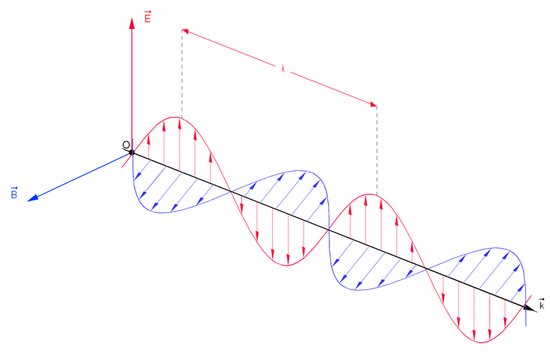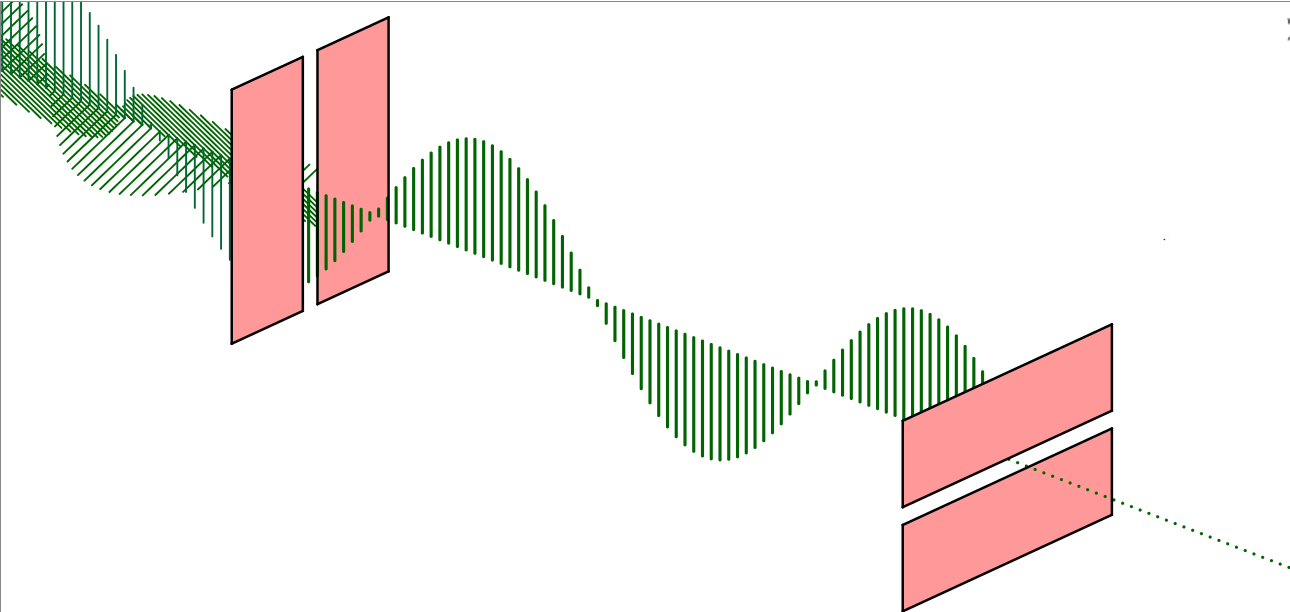QuestionAnswers

# Draw propagation diagram of a linearly polarized electromagnetic wave and write any two properties of electromagnetic waves.The amplitude of the magnetic field associated with the electromagnetic wave in vacuum is $B_{\circ}=50\times 10^{-8} Tesla$. Write the amplitude of the electric field in $Vm^{-1}$associated with the wave.Verified
129k+ views
Hint: What is a linearly polarized electromagnetic wave? All the electromagnetic waves have oscillating electric and magnetic fields in all directions, perpendicular to the line of propagation. But in linearly polarized or plane-polarized light, the direction of oscillation of these fields propagate only in a single plane. This can be achieved by placing a polaroid in the path of the traveling wave.
Formula used: $\vec E=\vec B\times \vec c$ where $c=3\times 10^8ms^{-1}$, is the speed of electromagnetic waves.The above picture is of a non-polarized electromagnetic wave. We can polarize it by placing a slit in its path:The above figure shows the two polaroid among which, the first one blocks all the oscillations except one. The second polaroid blocks all the remaining oscillations. Hence after crossing through the 2nd polaroid, the intensity of the wave drops to zero.
Two properties of electromagnetic waves:
A. Electromagnetic waves require no medium to travel.
B. In electromagnetic waves, there is an electric and magnetic field oscillating perpendicular to each other and mutually perpendicular to the line of propagation.
Given, Amplitude of magnetic field: $B_{\circ}=50\times 10^{-8} Tesla$
Now, using $\vec E=\vec B\times \vec c$
$E=50\times 10^{-8}\times 3\times 10^8=150Vm^{-1}$.
Hence the amplitude of electric field is $150\ Vm^{-1}$

Note:
Indeed, it is true that electromagnetic waves don’t require any medium to travel. It is because of the fact that the magnetic and electric field when propagated through free space creates their own medium in the free space. The speed of electromagnetic waves is the ultimate speed which equals $c=299,792,458 ms^{-1}$. Light is also an example of electromagnetic waves.